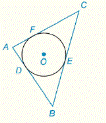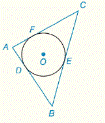Chapter 6.CR, Problem 34CRElementary Geometry For College St...

7th Edition
Alexander + 2 others
ISBN: 9781337614085

Solutions

Chapter
SectionElementary Geometry For College St...

7th Edition
Alexander + 2 others
ISBN: 9781337614085
Textbook Problem

G i v e n :                   ⊙ O   i s   i n s c r i b e d   i n   ∆ A B C                                                             A B = 9 ,   B C = 13 ,   A C = 10       F i n d :                           A D ,   B E ,   F CTo determine

To Find: AD, BE, FC if O is inscribed in ABC and AB=9, BC=13, AC=10.Explanation

Concept:

Theorem 6.3.4:

The tangent segments to a circle from an external point are congruent.

Calculation:

Given that O is inscribed in ABC and AB=9, BC=13, AC=10.

Let A,B, and C be vertices of the triangle.

AB,BC, and CA are the sides of the triangle.

Also, AB,BC,  and CA are the tangents of the circle at D, E and F respectively.

By Theorem 6.3.4:

The tangent segments to a circle from an external point are congruent.

Because AB=9, BC=13, AC=10

y+z=9

x+z=13

x+y=10

ABC is right triangle,

Still sussing out bartleby?

Check out a sample textbook solution.

See a sample solution

The Solution to Your Study Problems

Bartleby provides explanations to thousands of textbook problems written by our experts, many with advanced degrees!

Get Started

CHECK POINT 2. (a) W rite as radicals. (b)Write with fractional exponents:

Mathematical Applications for the Management, Life, and Social Sciences

Find all possible real solutions of each equation in Exercises 3144. x327=0

Finite Mathematics and Applied Calculus (MindTap Course List)

Sometimes, Always, or Never: If 0 ≤ an ≤ bn for all n and diverges, then converges.

Study Guide for Stewart's Single Variable Calculus: Early Transcendentals, 8th